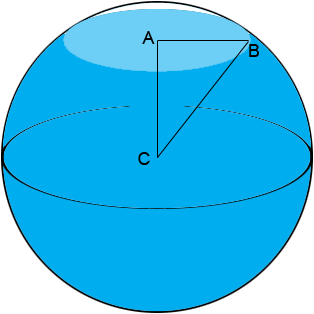Subject: spheres Name: lynne Who are you: Student I'd appreciate your help with this question: A spherical bowl of internal radius 15cm is partly filled with liquid. If the radius of the horizontal liquid surface is 12cm, calculate (taking the value of pi to be 3.142), the height of this liquid surface above the center of the bowl. Thank you! Hi Lynne, In the diagram C is the centre of the sphere, A is the centre of the circle that forms the horizontal liquid surface and B is a point on the surface of the liquid where it touches the sphere.The length of BC is 15 cm and the length of AB is 12 cm. What else do you know about the triangle ACB? How can you use this fact to find the length of AC? Penny